Circuit Elements & Signal Waveform

# Circuit Elements & Signal Waveform - Notes | Study Electrical Engineering SSC JE (Technical) - Electrical Engineering (EE)

 1 Crore+ students have signed up on EduRev. Have you?

Circuit Elements And Signal WaveForm

Introduction to Circuit Elements : Basic elements of networks are resistance, inductance and capacitance

Resistance : In network, the resistance is defined as per ohm's law.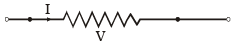V = R I
Constant of proportionality 'R' is termed as resistance.
R, depends upon the nature of material and also on its geometry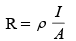where ρ →  resistivity, unit ohm-metre

I → length of wire, unit : metre

A → Area of cross section unit : m2

1/ρ = σ (conductivity)

unit of conductivity - slemens m–1 or mho m–1

• If length of wire is doubled and radius is half or halved, then resistivity remains same but resistance becomes 8 times.
• Extension of wire result into increase in length and decrease in area of cross-section therefore resistance of wire increases
• For compression resistance decreases.

Capacitance : The physical device that permits storage of charge is a capacitor and its ability to store charge is its capacitance. The reciprocal of capacitance is defined as elastance The charge Q is proportional to the potential difference V, i.e.
Q ∝ V

or Q = CV or C = Q/V

unit of capacitance is farad or coulombs/voit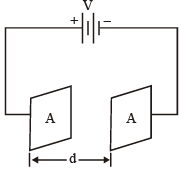The total energy required to transfer Q coulombs of charge, resulting in a final potential of V volts between the plates is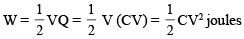Example : A parallel plate capacitor of 5pF capacitance has a charge of 0.1 μC on its plates. What is the energy stored in the capacitor?

(a) 1 mJ

(b) 1 μJ

(c) 1 nJ

(d) 1 pJ

Solution : (a)
Energy store = 1/2 VQ

= Q2/2C = 1mJ

• For the parallel plate capacitor shows above

C ∝ A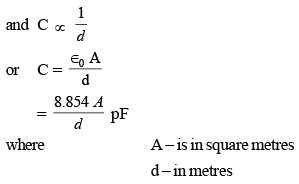The above equation is for vacuum, for any dielectric medium of dielectric constant ' ∈ ' in SI units.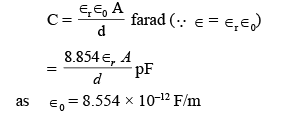t → relative permittivity or dielectric constant of the medium between the plates 

• For other geometric shapes such as two spheres two conductors, or one conductor and and ground, the formula would be different.

In each case C will be function of the geometry of the conductors and ∈ .

Inductance :

N → total no. of turns.
S → area of cross–section of coil

Then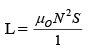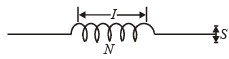where I → length of the coil

'L' is called the self inductance of the coil.

Relationship of parameters: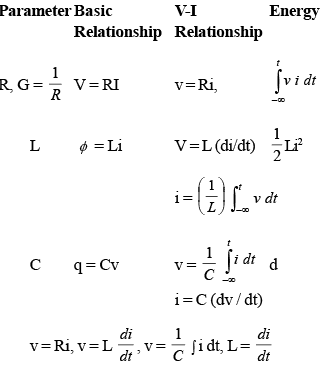Definitions: Before going into details of discussion of network analysis, it is desirable to introduce certain definitions.

Lumped and distributed Network

Lumped Network :A network in which we can separate resistors, capacitors and inductors physically.

Distributed Network : A circuit in which the voltage and current are functions of time and position is called, a distributed parameter circuit. While a circuit in which the voltage and current are functions of time only is called a lumped parameter circuit. 

• In distributed network, resistors, capacitors and inductors cannot be electrically separated and individually isolated as separate elements. Transmission line is such a network.

Active and Passive network

Passive Network : A network containing circuit elements without any energy sources.

Active Network : A network containing energy sources together with other circuit elements.

Linear Element :

A circuit element is linear if the relation between current and voltage involves a constant coefficient e.g.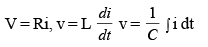• A linear network is one in which the principle of superposition (linearity test) holds, A nonlinear network is one which is not linear.

Average value : The general periodic function y (t) with period T has an averange value Yav given by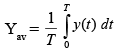RMS of effective value : The general function y(t), with period T has an effective value Yrms given by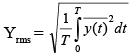• R.M.S. value of the functions asin ωt and acos ωt is a / √2

RMS value for several sine and cosine terms :

The function
y(t) = a0 + (a1 cos ωt + b2 sin 2 ωl) has an effective value given by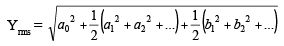also if A, is the effective value of a cos ωt then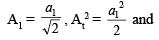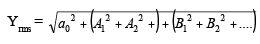Example : A series R–C circuit with R = 3 Ω and XC = 4 Ω at 50 Hz is supplied with a voltage V = 50 + 141 4sin 314 t. What isthe RMS value of the current flowing through the circuit?

(A) 5 A           (B) 10 A
(C) 20 A        (D) 22.36 A

Solution : (D) Impedance,

Z = R – jXC = 3 – j4 = 5 ∠ – 53.13°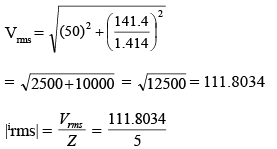= 22.36A

Definitions of Certain Terms

Node Any point in a circuit where the terminals of two or more elements are connected together.

Branch A branch is a part of the circuit which extends from one node to another A branch may contain one element or several elements in series. It has two terminals.

Essential Node If three or more elements are connected together at a node, then that node is sometimes called essential node.

Essential Branch A path which connects two essential nodes without passing through an essential node is called an essential branch.

Mesh Any path which contains no other paths within it is called a mesh.

Loop A path which contains more than two meshes is called a loop. Thus a loop contains meshes but a mesh does not contain a loop.

Kirchoff's Voltage Law (KVL) The algebraic sum of all branch voltages around any closed loop of a network is zero at all instants of time.
Mathematically ∑v(t ) = 0

• In most of the cases wer shall choose clockwise direction for traversing the closed path. 
• Also the voltage across elements that are traversed from – to + are taken positive and the voltages across elements that are traversed from + to – are taken negative (or you can choose vice-versa).

Illustration :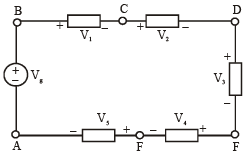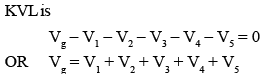Kirchoff's Current Law (KCL) At any instant of time, the algebraic sum of currents at a node is zero

Mathematically ∑i(t ) = 0

At nodes : Current entering → node are assigned positive sign

Current leaving → node, will assigned negative sign or vice versa

• Sign convention is arbitrary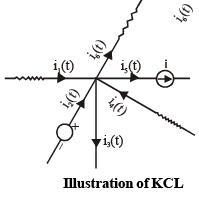Applying KCL at node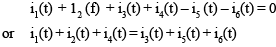Resistor in series :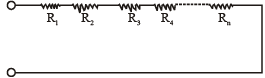Resistance in series and its equivalent
Req = R1 + R+ .....+ Rn

Resistances in parallel :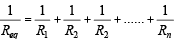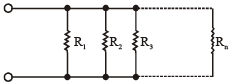Resistance in parallel and its equivalent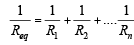Voltage Division Equations : Between two resistors in series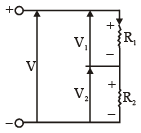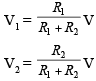Concept can be extended to 'n' series resistances

Current divider equations :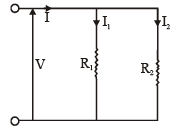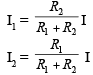• This concept can also be extended to 'n' parallel resistances.

Star ⇔ DELTA (OR T ⇔ π) Transformation :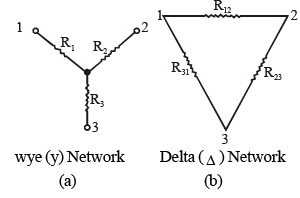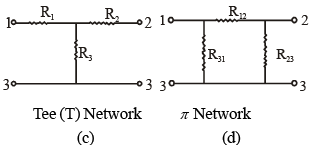(a) Delta to star (or π to T) Transoformation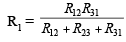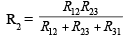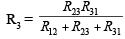(b) Star-to delta (or T to π ) Transformation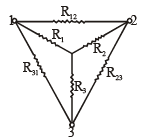Superimposed delta and star networks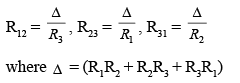Inductors in series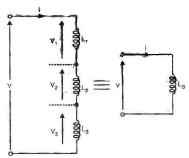Ls = L1 + L2 + L+ .............. + Ln

Inductors in parallel :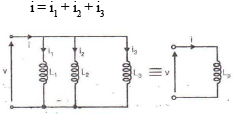Inductors in parallel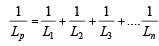Capacitors in series :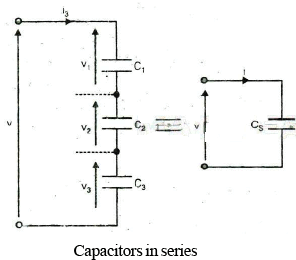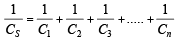Capacitors in parallel :

i = Cp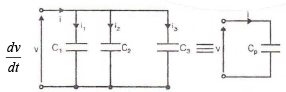CP = C1 + C2 + C3 + .... + Cn .

Source - Transformation :
a. voltage to current transformation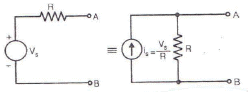b. current to voltage transformation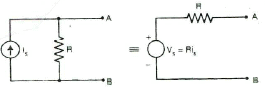The document Circuit Elements & Signal Waveform - Notes | Study Electrical Engineering SSC JE (Technical) - Electrical Engineering (EE) is a part of the Electrical Engineering (EE) Course Electrical Engineering SSC JE (Technical).
All you need of Electrical Engineering (EE) at this link: Electrical Engineering (EE)

## Electrical Engineering SSC JE (Technical)

2 videos|75 docs|37 tests
 Use Code STAYHOME200 and get INR 200 additional OFF

## Electrical Engineering SSC JE (Technical)

2 videos|75 docs|37 tests

Track your progress, build streaks, highlight & save important lessons and more!

,

,

,

,

,

,

,

,

,

,

,

,

,

,

,

,

,

,

,

,

,

;